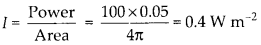Enlightened

# Question 12: NCERT Solutions for 12th Class Physics: Chapter 8-Electromagnetic Waves

• 0

Question 12: NCERT Solutions for 12th Class Physics: Chapter 8-Electromagnetic Waves

About 5% of the power of a 100 W light bulb is converted to visible radiation. What is the average intensity of visible radiation
(a) at a distance of 1 m from the bulb?
(b) at a distance of 10 m?
Assume that the radiation is emitted is o tropically and neglect reflection.

Share

1. Solution:
The bulb as a point source, radiate light in all direction uniformly and it is given that only 5% of power is converted to visible radiations.
(a) Let us assume a sphere of radius 1 m. Surface area A = 4πr2 = 4π (l)2 = 4 π m2 Intensity on the sphere(b) Let us assume a sphere of 10 m radius. Surface area A = 4π2 = 4K (10)2 = 400 π m2 Intensity on the sphereCheck the complete chapter with solutions.

NCERT Solutions for 12th Class Physics: Chapter 8-Electromagnetic Waves

• 0